# NCERT Solutions For Class 10 Maths Chapter 9 Exercise 9.1

Go back to  'Some Applications of Trigonometry'

## Chapter 9 Ex.9.1 Question 1

A circus artist is climbing a $$20\,\rm{m}$$ long rope, which is tightly stretched and tied from the top of a vertical pole to the ground. Find the height of the pole, if the angle made by the rope with the ground level is $$30^\circ$$ see Figure.

### Solution

What is Known?

(i) Length of rope $$=$$ $$20\,\rm{m}$$

(ii) Angle of rope with ground $$=$$ $$30^\circ$$$$=$$ $$\angle ACB$$

What is Unknown?

Height of pole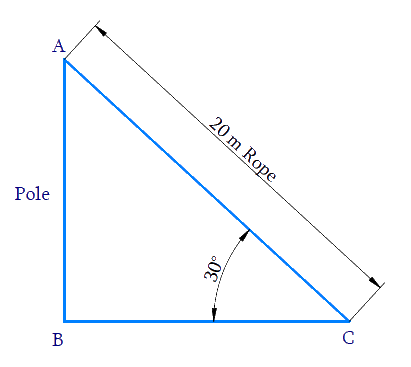Reasoning:

$$AB =$$ Height of the Pole.

$$BC =$$ Distance between the point on the ground and the pole.

$$AC =$$ Length of the Rope (Hypotenuse).

We need to find the height of the pole $$AB,$$ from the angle $$C$$ and the length of the rope $$AC.$$ Therefore, Trigonometric ratio involving all the three measures is $${\rm{sin}}\, C.$$

In $$\Delta ABC$$,

\begin{align} {AB} &=\frac{\text { opposite }}{\text { hypotenuse }} \\ {\rm{sin}}\,C &=\frac{{AB}}{{AC}} \\ {\rm{sin}}\, {C} &=\frac{{AB}}{20} \\ \frac{1}{2} &=\frac{{AB}}{20} \\ {AB}&=\frac{1}{\not{2}} \times \not\!\!{20}^{10}\ \\ {AB} &=10 \rm {m} \end{align}

Height of pole $$AB = 10\rm\,m.$$

## Chapter 9 Ex.9.1 Question 2

A tree breaks due to storm and the broken part bends so that the top of the tree touches the ground making an angle $$30^{\circ}$$ with it. The distance between the foot of the tree to the point where the top touches the ground is $$8\,\rm{m}$$. Find the height of the tree.

#### What is Known?

(i) Broken part of the tree bends and touching the ground making an angle of $$30^{\circ}$$ with the ground.

(ii) Distance between foot of the tree to the top of the tree is $$8\,\rm{m.}$$

#### What is Unknown?

Height of the tree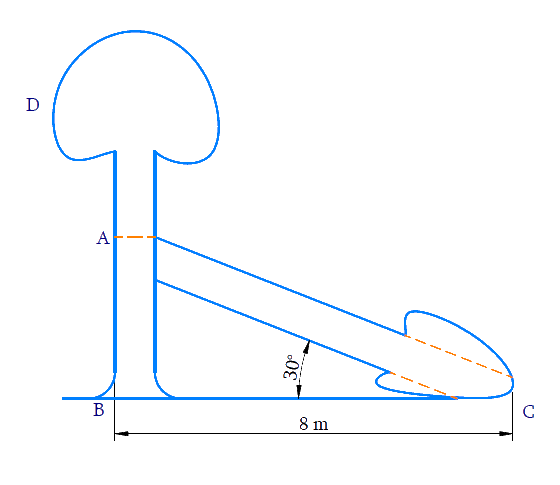#### Reasoning:

(i) Height of the tree $$= AB + AC.$$

(ii) Trigonometric ratio which involves $$AB, BC$$ and $$\angle C$$ is $$\tan \theta$$ , where $$AB$$ can be measured.

(iii) Trigonometric ratio which involves $$AB, AC$$ and $$\angle C$$ is $$\sin \theta$$ , where $$AC$$ can be measured.

(iv) Distance between the foot of the tree to the point where the top touches the ground $$= BC = 8\,\rm{ m}$$

#### Steps:

In $$\Delta ABC$$,

\begin{align}\tan C &=\frac{A B}{B C} \\ \tan 30^{\circ} &=\frac{A B}{8} \\ \frac{1}{\sqrt{3}} &=\frac{A B}{8} \\ A B &=\frac{8}{\sqrt{3}} \mathrm{m} \end{align}

\begin{align}\sin \,C{\rm{ }} &= {\rm{ }}\frac{{AB}}{{AC}}\\\sin 30^\circ &= \left( {\frac{{\frac{8}{{\sqrt 3 }}}}{{AC}}} \right)\\\frac{1}{2}{\rm{ }} &= {\rm{ }}\frac{8}{{\sqrt 3 }} \times \frac{1}{{AC}}\\AC{\rm{ }} &= {\rm{ }}\frac{8}{{\sqrt 3 }} \times 2\\AC{\rm{ }} &= {\rm{ }}\frac{{16}}{{\sqrt 3 }}\,\end{align}

\begin{align}\text{Height of tree}\, &= \rm{ }AB + AC\\&= \frac{8}{{\sqrt 3 }}+ \frac{{16}}{{\sqrt 3 }}\\&= \frac{{24}}{{\sqrt 3 }}\\&= \frac{{24}}{{\sqrt 3 }} \times \frac{{\sqrt 3 }}{{\sqrt 3 }}\\&= \frac{{24\sqrt 3 }}{3}\\&= 8\sqrt 3 \end{align}

Height of tree $$=8 \sqrt{3} \rm{m}$$

## Chapter 9 Ex.9.1 Question 3

A contractor plans to install two slides for the children to play in a park. For the children below the age of $$5$$ years, she prefers to have a slide whose top is at a height of $$1.5\,\rm{m}$$ and is inclined at an angle of $$30^\circ$$ to the ground, whereas for elder children she wants to have a steep slide at a height of $$3\,\rm{m}$$ and inclined at an angle of $$60^\circ$$ to the ground. What should be the length of the slide in each case?

### Solution

What is Known?

(i) For the children below the age of $$5$$ years.

Height of the slide $$=$$ $$1.5\,\rm{m}$$

Slide’s angle with the ground $$=$$ $$30^\circ$$

What is Unknown?

Length of the slide for children below the age of $$5$$ years and elder children.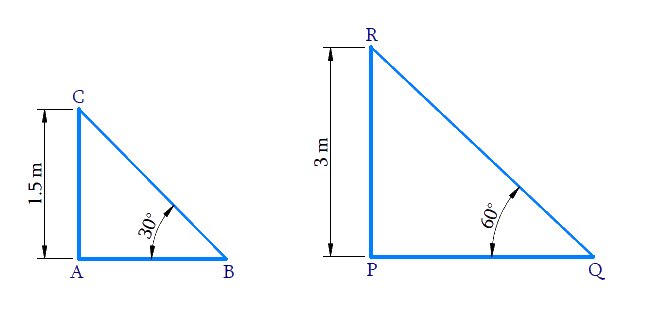(ii) For elder children.

Height of the slide $$= 3\,\rm{m}$$

Slide’s angle with the ground $$=$$$$60^\circ$$

Reasoning:

Let us consider the following conventions for the slide installed for children below $$5$$ years :

• The height of the slide as $$AC.$$
• Distance between the foot of the slide to the point where it touches the ground as $$AB.$$
• Length of the slide as $$BC.$$

Let us consider the following conventions for the slide installed for elder children:

• The height of the slide $$PR.$$
• Distance between the foot of the slide to the point where it touches the ground as $$PQ.$$
• Length of the slide as $$QR.$$

(i) Trigonometric ratio involving $$AC, BC$$ $$\angle B$$ and is $$\sin \theta$$

(ii) Trigonometric ratio involving $$PR, QR$$ and $$\angle Q$$ and is $$\sin \theta$$

Steps:

(i) In $$\Delta ABC$$,

\begin{align} \sin 30^{\circ} &=\frac{A C}{B C} \\ \frac{1}{2} &=\frac{1.5}{B C} \\ B C &=1.5 \times 2 \\ B C &=3 \mathrm{m}\end{align}

(ii) In $$\Delta \rm{PRQ}$$,

\begin{align}\sin \,Q &= \frac{{PR}}{{QR}}\\\sin \,60^\circ & = \frac{3}{{QR}}\\\frac{{\sqrt 3 }}{2}& = \frac{3}{{QR}}\\QR &= \frac{{3 \times 2}}{{\sqrt 3 }}\\&= \frac{6}{{\sqrt 3 }} \times \frac{{\sqrt 3 }}{{\sqrt 3 }}\\&= \frac{{6\sqrt 3 }}{3}\,\\&= 2\sqrt 3 \end{align}

Length of slide for children below $$5$$ years $$= 3\rm{m}.$$

Length of slide for elder children $$=$$ $$2 \sqrt3\,\rm{m}$$

## Chapter 9 Ex.9.1 Question 4

The angle of elevation of the top of a tower from a point on the ground, which is $$30\,\rm{m}$$ away from the foot of the tower, is $$30^\circ$$. Find the height of the tower.

### Solution

What is Known?

(i) Angle of elevation of the top of the tower from a point on ground is $$30^\circ$$

(ii) Distance between the foot of the tower to the point on the ground is $$30\,\rm{m.}$$

What is Unknown?

Height of the tower,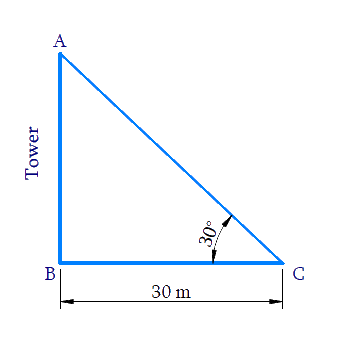Reasoning:

Let us consider the height of the tower as $$AB,$$ distance between the foot of tower to the point on ground as $$BC .$$

In $$\Delta ABC$$,

Trigonometric ratio involving $$AB, BC$$ and $$\angle C$$ is $$\tan \theta$$.

Steps:

In $$\Delta ABC$$,

\begin{align}\tan \,C &= \frac{{AB}}{{BC}}\\\tan 30^\circ &= \frac{{AB}}{{30}}\\\frac{1}{{\sqrt 3 }} &= \frac{{AB}}{{30}}\\AB &= \frac{{30}}{{\sqrt 3 }}\\&= \frac{{30}}{{\sqrt 3 }} \times \frac{{\sqrt 3 }}{{\sqrt 3 }}\\ &= \frac{{30\sqrt 3 }}{3}\\&= 10\sqrt 3 \end{align}

Height of tower $$AB$$ $$=10 \sqrt{3} \mathrm{m}$$

## Chapter 9 Ex.9.1 Question 5

A kite is flying at a height of $$60\,\rm{m}$$ above the ground. The string attached to the kite is temporarily tied to a point on the ground. The inclination of the string with the ground is $$60^\circ$$. Find the length of the string, assuming that there is no slack in the string.

### Solution

What is Known?

(i) Kite flying at a height $$= 60\,{\rm { m}}$$

(ii) Angle made by the string to the ground $$=60^\circ$$

What is Unknown?

Length of the string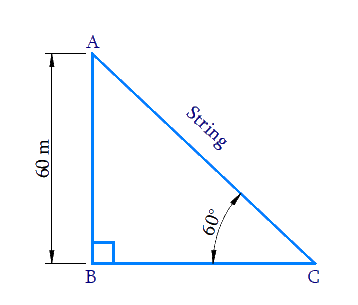Reasoning:

Let the height of the flying kite as $$AB$$, length of the string as $$AC$$ and the inclination of the string with the ground as $$\angle C$$

Trigonometric ratio involving $$AB, AC$$ and $$\angle C$$  is $$\sin \theta$$

Steps:

In $$\Delta ABC$$,

\begin{align}\sin \,C &= \frac{{AB}}{{AC}}\\\sin \,{60^0} &= \frac{{60}}{{AC}}\\\frac{{\sqrt 3 }}{2} &= \frac{{60}}{{AC}}\\AC &= \frac{{60 \times 2}}{{\sqrt 3 }}\\&= \frac{{120}}{{\sqrt 3 }} \times \frac{{\sqrt 3 }}{{\sqrt 3 }}\\&= \frac{{120\sqrt 3 }}{3}\\&= {\rm{40}}\sqrt {\rm{3}} \,\end{align}

Length of  the string $$AC =$$ $$40 \sqrt{3} \mathrm{m}$$

## Chapter 9 Ex.9.1 Question 6

A $$1.5\,\rm{m}$$ tall boy is standing at some distance from a $$30\,\rm{m}$$ tall building. The angle of elevation from his eyes to the top of the building increases from $$30^\circ$$ to $$60^\circ$$ as he walks towards the building. Find the distance he walked towards the building.

### Solution

What is Known?

(i) Height of the boy $$= 1.5\,{\rm{ m}}$$

(ii) Height of the building $$= 30\,{\rm {m}}$$

(iii) Angle of elevation from his eyes to the top of the building increases from $$30^\circ$$ to $$60^\circ$$ as he walks toward the building.

What is Unknown?

Distance, the boy walked towards the building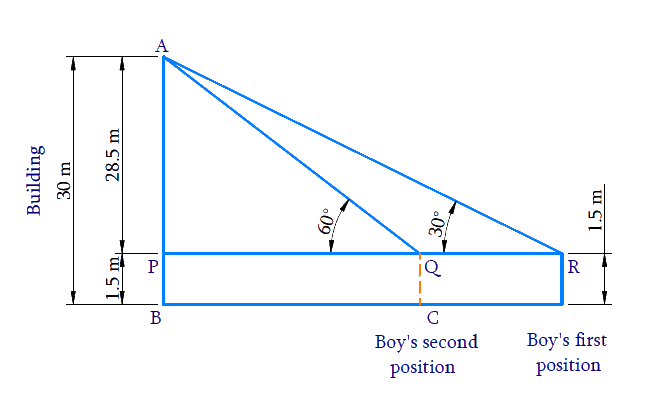Reasoning:

Trigonometric ratio involving ($$AP, PR$$ and $$\angle R$$) and ($$AP, PQ$$  and $$\angle Q$$) is $$tan \,\theta$$

[Refer thet  diagram to visualise $$AP, PR$$ and $$PQ$$]

Distance walked towards the building $$RQ$$ $$= PR – PQ$$

Steps:

In $$\Delta \mathrm{APR}$$

\begin{align} \tan R &=\frac{A P}{P R} \\ \tan 30^{\circ} &=\frac{28.5}{P R} \\ \frac{1}{\sqrt{3}} &=\frac{28.5}{P R} \\ P R &=28.5 \times \sqrt{3} \mathrm{m} \end{align}

In $$\Delta \mathrm{APQ}$$

\begin{align}{\tan \mathrm{Q}}&={\frac{A P}{P Q}} \\ {\tan 60^{\circ}}&={\frac{28.5}{P Q}}\\ \sqrt{3} &=\frac{28.5}{P Q} \\ P Q &=\frac{28.5}{\sqrt{3}} \mathrm{m} \end{align}

Therefore,

\begin{align}PR - PQ &= 28.5\sqrt 3 - \frac{{28.5}}{{\sqrt 3 }}\\ &= 28.5\left( {\sqrt 3 - \frac{1}{{\sqrt 3 }}} \right)\\&= 28.5\left( {\frac{{3 - 1}}{{\sqrt 3 }}} \right)\\&= 28.5\left( {\frac{2}{{\sqrt 3 }}} \right)\\&= \frac{{57}}{{\sqrt 3 }}\\&= \frac{{57}}{{\sqrt 3 }} \times \frac{{\sqrt 3 }}{{\sqrt 3 }}\\\rm&= \frac{{57\sqrt 3 }}{3}\\&= 19\sqrt 3 {\rm{m}}\end{align}

Distance the boy walked towards the building is $$19 \sqrt{3} \mathrm{m}$$.

## Chapter 9 Ex.9.1 Question 7

From a point on the ground, the angles of elevation of the bottom and the top of a transmission tower fixed at the top of a $$20\,\rm{m}$$ high building are $$45^\circ$$ and $$60^\circ$$ respectively. Find the height of the tower.

### Solution

What is Known?

(i) Angle of elevation from ground to bottom of the tower $$= 45^\circ$$

(ii) Angle of elevation from ground to top of the tower $$= 60^\circ$$

(iii) Height of the building $$= 20 \,\rm{m}$$

(iv) Tower is fixed at the top of the $$20\,\rm{m}$$ high building

What is Unknown?

Height of the tower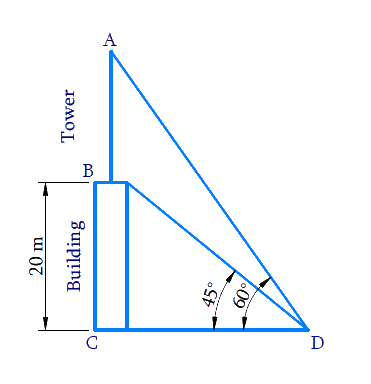Reasoning:

Let the height of the building is $$BC$$, height of the transmission tower which is fixed at the top of the building is $$AB$$. $$D$$ is the point on the ground from where the angles of elevation of the bottom $$B$$ and the top $$A$$ of the transmission tower $$AB$$ are $$45^\circ$$ and $$60^\circ$$ respectively.

The distance of the point of observation $$D$$ from the base of the building $$C$$ is $$CD$$

Combined height of the building and tower $$= AC = AB + BC$$

Trigonometric ratio involving sides $$AC,\, BC, \,CD$$ and $$∠D\, (45^\circ \rm{and}\, 60^\circ)$$ is $$\tan \theta$$

Steps:

In $$\Delta {BCD}$$

\begin{align}\tan 45^{\circ} &=\frac{B C}{C D} \\ 1 &=\frac{20}{C D} \\ C D &=20 \mathrm{m} \end{align}

In  $$\Delta {ACD}$$

\begin{align} \tan 60^{\circ} &=\frac{A C}{C D} \\ \sqrt{3} &=\frac{A C}{20} \\ A C &=20 \sqrt{3} \mathrm{m} \end{align}

Height of the tower,

$$AB = AC - BC$$

\begin{align}AB&=20\sqrt{3}\text m-20\text m \\ & =20\left( \sqrt{3}-1 \right)\rm m \end{align}

## Chapter 9 Ex.9.1 Question 8

A statue, $$1.6\,\rm{m}$$ tall, stands on the top of a pedestal, from a point on the ground. The angle of elevation of the top of the statue is $$60^\circ$$ and from the same point the angle of elevation of the top of the pedestal is $$45^\circ$$. Find the height of the pedestal.

### Solution

What is Known?

(i) Height of statue $$= 1.6 \,\rm{m}$$

(ii) Angle of elevation from ground to top of the statue $$= 60^\circ$$

(iii) Statue stands on the top of the pedestal.

(iv) Angle of elevation of the top of the pedestal (bottom of the statue) $$= 45^\circ$$

What is Unknown?

Height of the pedestal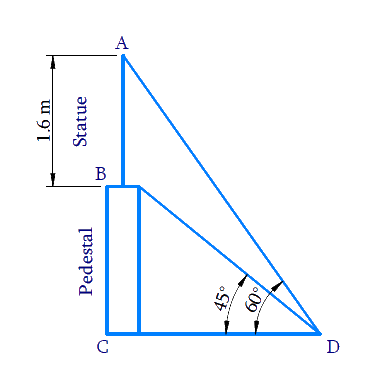Reasoning:

Let the height of the pedestal is $$BC$$, height of the statue, stands on the top of the pedestal, is $$AB$$. $$D$$ is the point on the ground from where the angles of elevation of the bottom $$B$$ and the top $$A$$ of the statue $$AB$$ are $$45^\circ$$ and $$60^\circ$$respectively.

The distance of the point of observation $$D$$ from the base of the pedestal $$C$$ is $$CD$$

Combined height of the pedestal and statue $$AC = AB + BC$$

Trigonometric ratio involving sides $$AC,\, BC, \,CD$$ and $$∠D$$ ($$45^\circ$$and $$60^\circ$$) is $$\tan \theta$$

Steps:

In $$\Delta BCD$$,

\begin{align}\tan {45^0} &= \frac{{BC}}{{CD}}\\1 &= \frac{{BC}}{{CD}}\\BC &= CD\quad\dots \left( {\rm{i}} \right)\end{align}

In  $$\Delta {ACD}$$

\begin{align}\tan {\rm{ }}{60^0} &= \frac{{AC}}{{CD}}\\\tan {\rm{ }}{60^0} &= \frac{{AB + BC}}{{CD}}\\\sqrt 3 &\!=\!\!\frac{{1.6 + BC}}{{BC}}\left[ {{\rm{from}}\left( {\rm{i}} \right)} \right]\\\sqrt 3 BC &= 1.6 + BC\\\sqrt 3 BC - BC &= 1.6\\BC\left( {\sqrt 3 - 1} \right) &= 1.6\\BC = \frac{{1.6}}{{\sqrt 3 - 1}} &\times \frac{{\sqrt 3 + 1}}{{\sqrt 3 + 1}}\\&= \frac{{1.6\left( {\sqrt 3 + 1} \right)}}{{3 - 1}}\\&= \frac{{1.6\left( {\sqrt 3 + 1} \right)}}{2}\\&= 0.8\left( {\sqrt 3 + 1} \right)\end{align}

Height of pedestal $$BC$$ $$=0.8(\sqrt{3}+1) \mathrm{m}$$

## Chapter 9 Ex.9.1 Question 9

The angle of elevation of the top of a building from the foot of the tower is $$30^\circ$$ and the angle of elevation of the top of the tower from the foot of the building is $$60^\circ.$$ If the tower is $$50\,\rm{m}$$ high, find the height of the building.

### Solution

What is Known?

(i) Angle of elevation of the top of a building from the foot of the tower  $$=30^\circ$$

(ii) Angle of elevation of the top of the tower from the foot of the building $$=60^\circ$$

(iii) Height of tower $$= 50\,{\rm{m}}$$

What is  Unknown?

Height of the buildingReasoning:

Let the height of the tower is $$AB$$ and the height of the building is $$CD$$. The angle of elevation of the top of the building $$D$$ from the foot of the tower $$B$$ is $$30^\circ$$ and the angle of elevation of the top of the tower $$A$$ from the foot of the building $$C$$ is $$60^\circ$$.

Distance between the foot of the tower and the building is $$BC$$.

Trigonometric ratio involving sides $$AB, \,CD, \,BC$$ and angles $$∠B$$ and $$∠C$$ is $$\tan \theta$$

Steps:

In  $$\Delta ABC$$

\begin{align}{\text{tan 6}}{{\rm{0}}^0} &= \frac{{AB}}{{BC}}\\\sqrt 3 &= \frac{{50}}{{BC}}\\BC &= \frac{{50}}{{\sqrt 3 }}\quad\dots\left( {\rm{i}} \right)\end{align}

In $$\Delta BCD$$,

\begin{align} \tan {{30}^{0}}&=\frac{CD}{BC} \\ \frac{1}{\sqrt{3}}&=\frac{CD}{BC} \\ \frac{1}{\sqrt{3}}&=\frac{CD}{\frac{50}{\sqrt{3}}}\qquad\left[ \text{from}\left( \text{i} \right) \right] \\ CD&=\frac{1}{\sqrt{3}}\times \frac{50}{\sqrt{3}} \\ CD&=\frac{50}{3} \\ CD&=16\frac{2}{3} \end{align}

Height of the building \begin{align}C D=16 \frac{2}{3} \mathrm{m}\end{align}

## Chapter 9 Ex.9.1 Question 10

Two poles of equal heights are standing opposite each other on either side of the road, which is $$80\,\rm{m}$$ wide. From a point between them on the road, the angles of elevation of the top of the poles are $$60^\circ$$ and $$30^\circ$$ respectively. Find the height of the poles and the distances of the point from the poles.

### Solution

What is Known?

(i) The poles of equal height.

(ii) Distance between poles $$= 80\,\rm{m}$$

(iii) From a point between the poles, the angle of elevation of the top of the poles are $$60^\circ$$ and $$30^\circ$$ respectively.

#### What is Unknown?

Height of the poles and the distances of the point from the poles.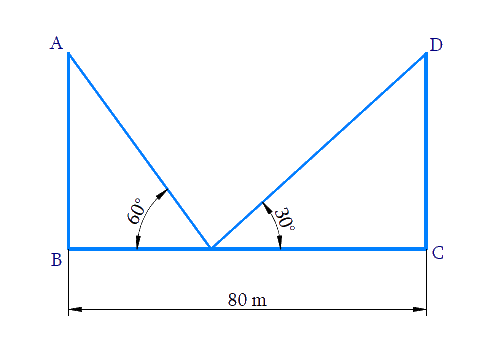Reasoning:

Let us consider the two poles of equal heights as $$AB$$ and $$DC$$ and the distance between the poles as $$BC$$. From a point $$O$$, between the poles on the road, the angle of elevation of the top of the poles $$AB$$ and $$CD$$ are $$60^\circ$$ and $$30^\circ$$ respectively.

Trigonometric ratio involving angles, distance between poles and heights of poles is $$\tan \theta$$

Steps:

Let the height of the poles be $$x$$

Therefore $$AB=DC=x$$

In $$\Delta AOB,$$

\begin{align}\text{tan 6}{{\text{0}}^{0}}&=\frac{AB}{BO} \\ \sqrt{3}&=\frac{x}{BO} \\ BO&=\frac{x}{\sqrt{3}}\quad\dots\left( \text{i} \right) \end{align}

In $$\Delta OCD,$$

\begin{align}& \tan \text{ }{{30}^{0}}=\frac{DC}{OC} \\& \frac{1}{\sqrt{3}}=\frac{x}{BC-BO} \\ & \frac{1}{\sqrt{3}}=\frac{x}{80-\frac{x}{\sqrt{3}}}\qquad\left[ \text{from }\left( \text{i} \right) \right] \\ & 80-\frac{x}{\sqrt{3}}=\sqrt{3}x \\ & \frac{x}{\sqrt{3}}+\sqrt{3}x=80 \\ & x\left( \frac{1}{\sqrt{3}}+\sqrt{3} \right)=80 \\ & x\left( \frac{1+3}{\sqrt{3}} \right)=80 \\ & x\left( \frac{4}{\sqrt{3}} \right)=80 \\ & x=\frac{80\sqrt{3}}{4} \\ & x=20\sqrt{3} \\ \end{align}

Height of the pole $$x = 20\sqrt 3 {\rm{ }}\,\rm m$$

Distance of point from $$O$$ the poles $$AB$$

\begin{align} BO&=\frac{x}{\sqrt{3}} \\ & =\frac{20\sqrt{3}}{\sqrt{3}} \\ & =20 \end{align}

Distance of point from $$O$$ the poles $$CD$$

\begin{align}OC&=BC-BO \\ & =80-20 \\ & =60 \end{align}

Height of the poles are  $$20\sqrt{3}\text{ }\rm{m}$$  and the distances of the point from the poles are $$20\rm{m}\text{ and }60\rm{m}$$ .

## Chapter 9 Ex.9.1 Question 11

A TV tower stands vertically on the bank of a canal. From a point on the other bank directly opposite the tower, the angle of elevation of the top of the tower is $$60^\circ$$. From another point $$20 \,\rm{m}$$ away from this point on the line joining this point to the foot of the tower, the angle of elevation of the top of the tower is $$30^\circ$$ see Figure. Find the height of the tower and the width of the canal.

### Solution

What is Known?

(i) The angle of elevation of the top of the tower from a point on the other bank directly opposite the tower is $$60^\circ$$

(ii) From another point $$20\,\rm{ m}$$ away from this point in (i) on the line joining this pointto the foot of the tower, the angle of elevation of the top of the tower is $$30^\circ$$

(iii)  $$CD =20\,\rm{ m}$$

What is Unknown?

Height of the tower $$=AB$$  and the width of canal $$=BC$$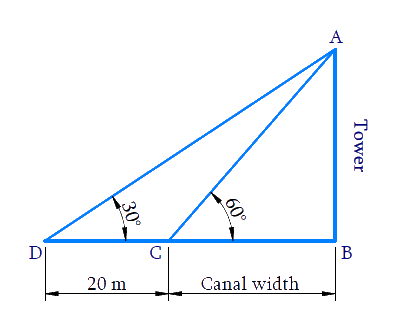Reasoning:

Trigonometric ratio involving $$CD,\, BC,$$ angles and height of tower $$AB$$ is $$tan\,\theta$$.

Steps:

Considering $$\Delta ABC,$$

\begin{align} \tan 60 ^ { \circ } & = \frac { A B } { B C } \\ \sqrt { 3 } & = \frac { A B } { B C } \\ A B & = \sqrt { 3 } B C \ldots . ( 1 ) \end{align}

Considering $$\Delta ABD,$$

\begin{align}\text{tan 3}{{\text{0}}^{0}}&=\frac{AB}{BD} \\ \text{tan 3}{{\text{0}}^{0}}&=\frac{AB}{CD+BC} \\ \frac{1}{\sqrt{3}}&\!\!=\!\!\frac{BC\sqrt{3}\,}{20+BC}{From}\,(1) \\ 20+BC&=BC\sqrt{3}\times \sqrt{3} \\ 20+BC&=3BC \\ 3BC-BC&=20 \\ 2BC&=20 \\ BC&=10\end{align}

Substituting$$BC = 10 \,\rm{m}$$ in Equation ($$1$$), we get

$$\text{AB = 10}\sqrt{\text{3}}\,\rm{m}$$

Height of the tower $$\text{AB = 10}\sqrt{\text{3}}$$

Width of the canal $$BC=10 \,\rm{m}$$

## Chapter 9 Ex.9.1 Question 12

From the top of a $$7 \,\rm{m}$$ high building, the angle of elevation of the top of a cable tower is $$60^\circ$$ and the angle of depression of its foot is $$45^\circ$$. Determine the height of the tower.

### Solution

What is Known?

(i) Height of building $$=7 \,\rm{m}$$

(ii) Angle of elevation of the top of a cable tower from building top $$=60^\circ$$

(iii) Angle of depression of the foot of the cable tower from building top $$= 45^\circ$$

What is Unknown?

Height of the tower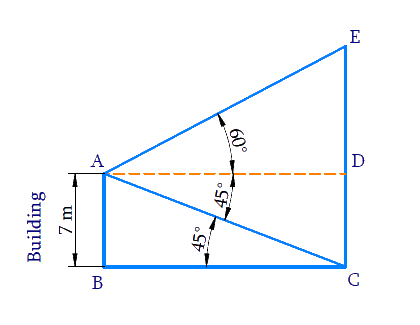Reasoning:

Let the height of the tower is $$CE$$ and the height of the building is $$AB$$. The angle of elevation of the top $$E$$ of the tower from the top $$A$$ of the building is $$60^\circ$$ and the angle of depression of the bottom $$C$$ of the tower from the top $$A$$of the building is $$45^\circ$$. Trigonometric ratio involving building height, tower height, angles and distances between them is $$\tan \theta$$

Steps:

Draw $$AD||BC$$.

Then,$$\angle DAC=\angle ACB={{45}^{0}}$$(alternate interior angles.)

In $$\Delta ABC$$,

\begin{align}\tan {{45}^{0}}&=\frac{AB}{BC} \\ 1&=\frac{7}{BC} \\ BC&=7 \end{align}

$$ABCD$$ is a rectangle,

Therefore, $$BC = AD = 7 \, \rm{m}$$ and $$AB = CD = 7 \, \rm{m}$$

In $$\Delta ADE$$,

\begin{align} { \tan 60 ^ \circ = \frac { E D } { A D } } \\ { \sqrt { 3 } = \frac { E D } { 7 } } \\ { E D = 7 \sqrt { 3 } } \end{align}

Height of tower

\begin{align}CE&=ED+CD \\ &=7\sqrt{3}+7 \\ &=7(\sqrt{3}+1) \end{align}

Height of the tower $$= 7 ( 1 + \sqrt { 3 } ) \,\rm{m}$$

## Chapter 9 Ex.9.1 Question 13

As observed from the top of a $$75\,\rm{m}$$ high lighthouse from the sea-level, the angles of depression of two ships are $$30^\circ$$ and $$45^\circ.$$ If one ship is exactly behind the other on the same side of the lighthouse, find the distance between the two ships.

### Solution

What is Known?

(i) Height of the lighthouse $$=75\,\rm{m}$$

(ii) Angles of depression of two ships from the top of the lighthouse are $$30^\circ$$ and $$45^\circ.$$

What is Unknown?

Distance between the two ships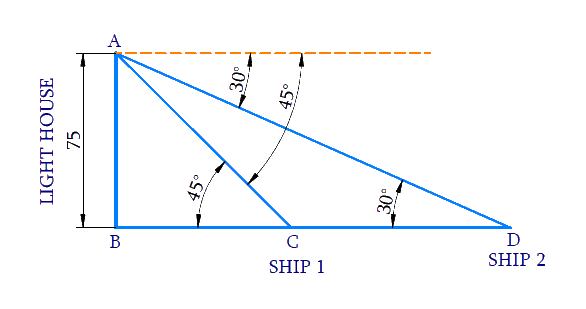Reasoning:

Let the height of the lighthouse from the sea-level is $$AB$$ and the ships are $$C$$ and $$D$$. The angles of depression of the ships $$C$$ and $$D$$ from the top $$A$$ of the lighthouse, are $$45^\circ$$ and $$60^\circ$$ respectively.

Trigonometric ratio involving $$AB, BC, BD$$ and angles is tan$$\theta$$.

Distance between the ships, $$CD = BD − BC$$

Steps:

In $$\Delta ABC$$,

\begin{align} \tan {{45}^{0}}&=\frac{AB}{BC} \\ 1&=\frac{75}{BC} \\ BC&=75\, \end{align}

In $$\Delta ABD$$,

\begin{align} \tan {{30}^{0}}&=\frac{AB}{BD} \\ \frac{1}{\sqrt{3}}&=\frac{75}{BD} \\ BD&=75\sqrt{3} \end{align}

Distance between two ships

$$CD=BD-BC$$

\begin{align}CD&=75\sqrt{3}-75 \\ &=75\left( \sqrt{3}-1 \right) \end{align}

Distance between two ships $$C D = 75 ( \sqrt { 3 } - 1 ) \, \rm{m}$$

## Chapter 9 Ex.9.1 Question 14

A $$1.2 \,\rm{m}$$ tall girl spots a balloon moving with the wind in a horizontal line at a height of $$88.2\,\rm{m}$$  from the ground. The angle of elevation of the balloon from the eyes of the girl at any instant is $$60^\circ.$$ After some time, the angle of elevation reduces to $$30^\circ$$ see Figure. Find the distance travelled by the balloon during the interval.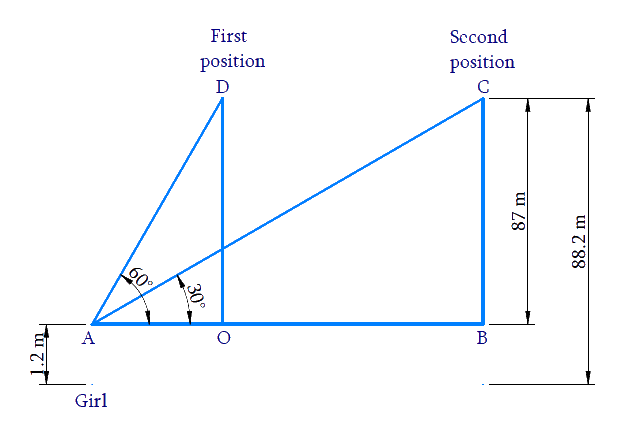### Solution

What is Known?

(i) Height of the girl $$=$$ $$1.2\,\rm{m}$$

(ii) Vertical height of balloon from ground $$=$$ $$88.2\,\rm{m}$$

(iii) Angle of elevation of the balloon from the eyes of the girl is reducing from $$60^\circ$$ to $$30^\circ$$ as the balloon moves along wind.

(iv) From the figure, $$OD = BC$$ can be calculated as

$$88.2\,\rm{m}$$ $$–$$ $$1.2\,\rm{m}$$ $$=$$ $$87\,\rm{m}\dots$$  Result (1)

What is Unknown?

Distance traveled by the balloon, $$OB$$

Reasoning:

Trigonometric ratio involving $$AB,\, BC,\, OD,\,OA$$ and angles is tan$$\theta$$. [Refer $$AB,\, BC,\, OA$$ and $$OD$$ from the figure.]

Distance travelled by the balloon $$OB = AB − OA$$

Steps:

From the figure, $$OD = BC$$, can be calculated as

$$88.2\rm {m}\, – 1.2 \rm {m} = 87 {m}$$---------------- ($$1$$)

In $$\Delta AOD,$$

\begin{align}\tan {{60}^{0}}&=\frac{OD}{OA} \\ \sqrt{3}&=\frac{87}{OA} \\ OA&=\frac{87}{\sqrt{3}} \\ &=\frac{87}{\sqrt{3}}\times \frac{\sqrt{3}}{\sqrt{3}} \\ &=\frac{87\times \sqrt{3}}{3} \\& =29\sqrt{3}\text{m} \end{align}

In $$\Delta ABC,$$

\begin{align}\tan {{30}^{0}}&=\frac{BC}{AB} \\ \frac{1}{\sqrt{3}}&=\frac{87}{AB} \\ AB&=87\sqrt{3} \end{align}

Distance traveled by the balloon $$OB = AB \,– OA$$

\begin{align}OB & =87\sqrt{3}-29\sqrt{3} \\ & =58\sqrt{3} \end{align}

Distance traveled by the balloon $$= 58 \sqrt { 3 } \, \,\rm{m}$$

## Chapter 9 Ex.9.1 Question 15

A straight highway leads to the foot of a tower. A man standing at the top of the tower observes a car at an angle of depression of $$30^\circ$$, which is approaching the foot of the tower with a uniform speed. Six seconds later, the angle of depression of the car is found to be $$60^\circ.$$ Find the time taken by the car to reach the foot of the tower from this point.

### Solution

What is Known?

(i) Angle of depression is $$30^\circ$$

(ii) $$6$$ seconds later angle of depression is $$60^\circ$$

What is Unknown?

Time taken by the car to reach the foot of the tower $$= CD$$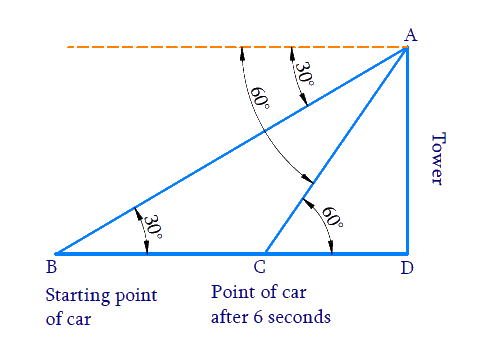Reasoning:

Let the height of the tower as $$AD$$ and the starting point of the car as $$B$$ and after $$6$$ seconds point of the car as $$C$$. The angles of depression of the car from the top $$A$$ of the tower at point $$B$$ and $$C$$ are $$30^\circ$$ and $$60^\circ$$ respectively.

Distance travelled by the car from the starting point towards the tower in $$6$$ seconds $$= BC$$

Distance travelled by the car after 6 seconds towards the tower $$= CD$$

Trigonometric ratio involving $$AD, \,BC, \,CD$$ and angles is $$tan\,\theta$$.

We know that,

$\text {Speed} = \frac { \text { Distance } } { \text { Time } }$

The speed of the car is calculated using the distance $$BC$$ and time $$= 6\,\rm seconds.$$ seconds. Using Speed and Distance $$CD,$$ time to reach foot can be calculated.

Steps:

In $$\Delta ABD$$,

\begin{align}\tan {{30}^{0}}&=\frac{AD}{BD} \\ \frac{1}{\sqrt{3}}&=\frac{AD}{BD} \\BD&=AD\sqrt{3}\,\,\,....(1) \end{align}

In $$\Delta ACD$$,

\begin{align} \tan {{60}^{0}}&=\frac{AD}{CD} \\ \sqrt{3}&=\frac{AD}{CD} \\ AD&=CD\sqrt{3}\,\,\,....(2)\text{ } \end{align}

From equation ($$1$$) and ($$2$$)

\begin{align}BD&=CD\sqrt{3}\times \sqrt{3} \\ BC+CD&=3CD\\&\left[ \because BD=BC+CD \right] \\ BC&=2CD\,\,\,\dots(3)\end{align}

Distance travelled by the car from the starting point towards the tower in $$6$$ seconds $$= BC$$ Speed of the car to cover distance $$BC$$ in $$6$$ seconds;

\begin{align}\text Speed&=\,\frac{\text{Distance}}{\text{Time}} \\ & =\,\frac{BC}{6\,} \\ &=\,\frac{2CD}{6}\,\qquad\left[ \text{from(3)} \right] \\ & =\,\frac{CD}{3}\, \end{align}

Speed of the car =\,\begin{align}\frac{CD}{3}\,\rm{m/s}\end{align}

Distance travelled by the car from point $$C$$, towards the tower $$= CD$$

Time to cover distance $$CD$$ at the speed of =\,\begin{align}\frac{CD}{3}\,\rm{m/s}\end{align}

\begin{align}\text Time &=\frac{\text{Distance}}{\text{Speed}} \\ & =\frac{CD}{\frac{CD}{3}}\, \\ & =CD\times \frac{3}{CD} \\ & =3 \end{align}

Time taken by the car to reach the foot of the tower from point $$C$$ is $$3$$ seconds.

## Chapter 9 Ex.9.1 Question 16

The angles of elevation of the top of a tower from two points at a distance of $$4\,\rm{m}$$  and $$9\,\rm{m}$$  from the base of the tower and in the same straight line with it are complementary. Prove that the height of the tower is $$6\,\rm{m.}$$

### Solution

What is Known?

Angle of elevation of the top of a tower from two points at a distance of $$4\,\rm{m}$$ and $$9\,\rm{m}$$ from the base of the tower are complimentary.

What is Unknown?

To prove height of the tower is $$6\,\rm{m.}$$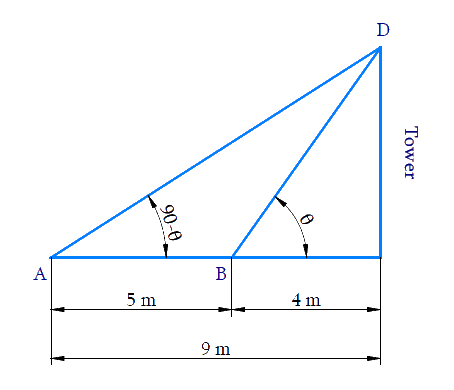Reasoning:

Let the height of the tower as $$CD$$. B is a point $$4\rm{m}$$ away from the base $$C$$ of the tower and $$A$$ is a point $$5\rm{m}$$ away from the point $$B$$ in the same straight line. The angles of elevation of the top $$D$$ of the tower from the points $$B$$ and $$A$$ are complementary.

Since the angles are complementary, if one angle is $$\theta$$ and the other is ($$90^\circ$$− $$\theta$$). Trigonometric ratio involving $$CD, \,BC,\, AC$$ and angles is $$tan\,\theta$$.

Using $$tan\,\theta$$ and tan( $$90^\circ$$ − $$\theta$$) $$=$$ $$cot\,\theta$$ ratios are equated to find the height of the tower.

Steps:

In $$\Delta BCD$$,

\begin{align}& \text{tan}\theta \,\text{= }\frac{CD}{BC} \\ & \text{tan}\theta \,\text{= }\frac{CD}{4}\quad\dots (1) \\ \end{align}

Here,

\begin{align}AC&=AB+BC \\ & =5+4 \\ & =9 \end{align}

In $$\Delta ACD$$,

\begin{align}{\rm{tan}}\left( {90 - \theta } \right)\,&= \frac{{CD}}{{AC}}\\\cot \theta &= \frac{{CD}}{9}\\&\left[ {\,{\rm{tan}}\left( {90 - \theta } \right)\, = \,\cot \theta } \right]\\\frac{1}{{{\rm{tan}}\theta }}\, &= \frac{{CD}}{9}\\&\left[ {\cot \theta = \frac{1}{{{\rm{tan}}\theta }}} \right]\\{\rm{tan}}\theta &= \frac{9}{{CD}}\quad \dots(2)\end{align}

From equation ($$1$$) and ($$2$$)

\begin{align}\frac{{CD}}{4} &= \frac{9}{{CD}}\\C{D^2} &= 36\\CD &= \pm 6\end{align}

Since, Height cannot be negative

Therefore, height of the tower is $$6\,\rm{m.}$$

Download SOLVED Practice Questions of NCERT Solutions For Class 10 Maths Chapter 9 Exercise 9.1 for FREE
Ncert Class 10 Exercise 9.1
Ncert Solutions For Class 10 Maths Chapter 9 Exercise 9.1

Related Sections
Related Sections
Instant doubt clearing with Cuemath Advanced Math Program